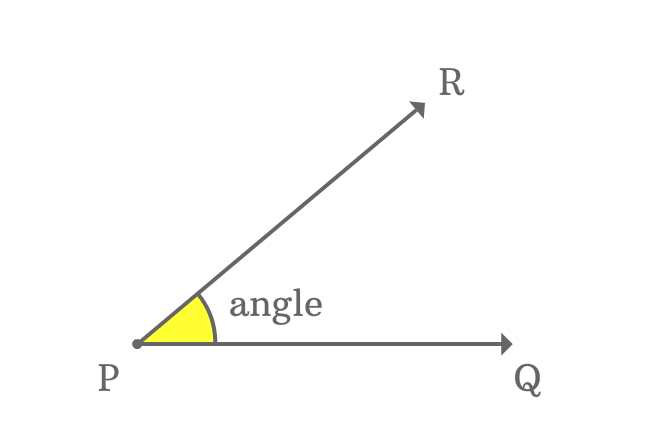# Representation of an angle

A system of expressing an angle in mathematical form is called the representation of an angle.

## Example

An angle is represented mathematically in a special format.$\overrightarrow{PQ}$ and $\overrightarrow{PR}$ are two lines and they formed an angle at their vertex $P$.

A special symbol ($\angle$) is used to represent an angle.

Angle is formed at point $P$ by the lines $\overrightarrow{PQ}$ and $\overrightarrow{PR}$. So, the end points of the arms of angle $\overrightarrow{PQ}$ and $\overrightarrow{PR}$ are written both sides of the vertex, followed by the angle symbol.

The angle is represented by either $\angle QPR$ and read as $angle \, QPR$. Similarly, it is also represented as $\angle RPQ$, read as $angle \, RPQ$.

Latest Math Topics
Jun 26, 2023
Jun 23, 2023

###### Math Questions

The math problems with solutions to learn how to solve a problem.

Learn solutions

Practice now

###### Math Videos

The math videos tutorials with visual graphics to learn every concept.

Watch now

###### Subscribe us

Get the latest math updates from the Math Doubts by subscribing us.